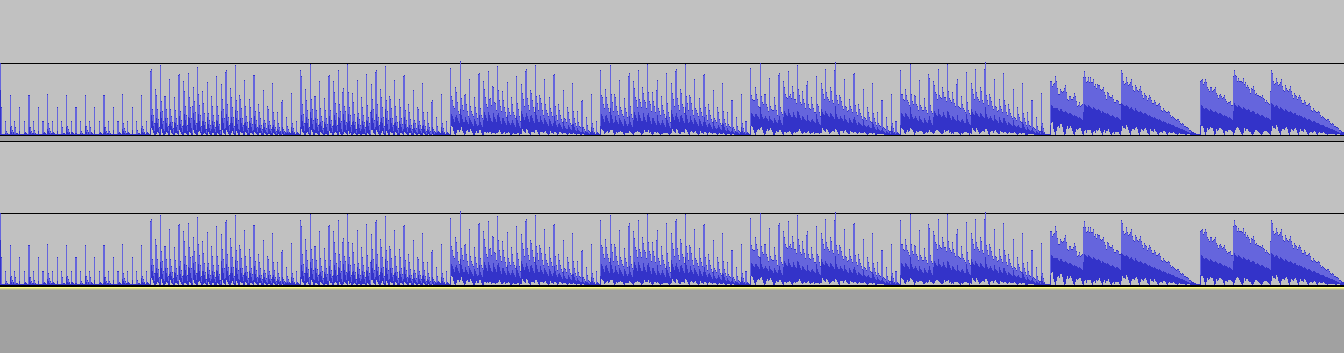# Making of the music (part 2) by TomCat

## PC-Speaker softsynth

### Sample

To make the PC speaker work like a PWM device, we set the PIT's channel #2 to operating mode zero (interrupt-on-terminal-count).

``` MOV AL,90H
OUT 43H,AL
```

Then we can output a 6-bit sample. Value 0 will be the silence, value 63 is the highest volume.

```IRQ:
PUSHA
MOV AL,2   ; Sample [0...127]
SHR AL,1   ; scaled to 6-bit
JZ .1      ; zero means pause (no sound)
OUT 42H,AL ; out the 6bit sample
.1:
...
POPA
IRET
```

### Tempo and notes

We set the timer counter to value 68 (later on called Divider), because we want 129 bpm for tempo.

``` MOV AL,68
OUT 40H,AL
MOV AL,0
OUT 40H,AL
```

Then the player frequency will be 17,5 kHz.
Hz=105000000/88/Divider,
bpm(low)=Hz*60sec/2/2/2/2/2/2/2/2/2/2/2/2/2/2,
bpm(high)=Hz*60sec/2/2/2/2/2/2/2/2/2/2/2/2/2.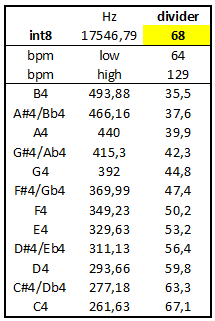To play musical notes, we need to multiply the carrier wave by some constant values. We can compute these values depending on the frequencies, for example the A4 note computed like this: A_4=(32*256*44000/Hz+50)/100.
I have an inlcude file for notes: notes.inc

### Drums

We had great fun with pc-speaker softsynth drums in a prod released earlier.
And this very simple drum pattern (bd - ht - sn/bd - ht) was used in another intro too, but with a different tempo: qblove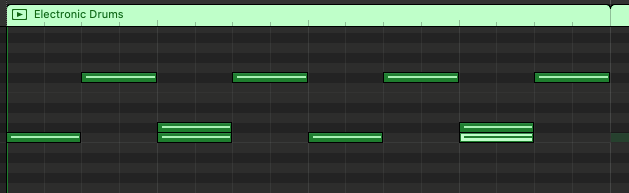Bass drum is a simple division: A constant value divided by the time (or timer counter).

```KICKDRUM:
MOV AX,16128   ; constant value
CWD            ; extend the value to 2 words
MOV CX,8191    ; get some lower bits of
AND CX,SI      ; the timer counter (SI)
INC CX         ; avoiding division by zero
DIV CX         ; get one bit only
AND AL,64      ; 0 or 64 will be the volume (the sample)
```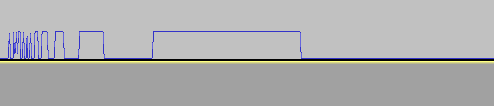Comparing an SN (on the left) to a BD (on the right), the snare is much longer, and more random.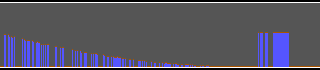Hihat also needs some random noise, so HT and SN starts with the same code snippet.

``` XCHG AX,DI    ; DI: pointer to video memory, which is quite random here :)
MUL SI        ; SI: the timer counter
XCHG AX,DX    ; AX: random number depending on time
AND AX,32     ; get only one random bit
```

### Chords

One chord consists of four summed notes.

``` MOV BP,NOTES
CHORDS:
SALC            ; AL: zero
MOV AH,[BP+DI]  ; AX: pitch F#, ...
MUL SI          ; SI: the timer counter
AND DX,1FH      ; DX: get a saw wave sample
ADD BH,DL       ; BH: sample (sum of the notes)
INC DI          ; next note
TEST DI,3       ; loop 4x
JNZ CHORDS
```

First, the idea of making a cover of a popular song in a restricted environment is great fun. That is why the length of the notes is other than power of 2.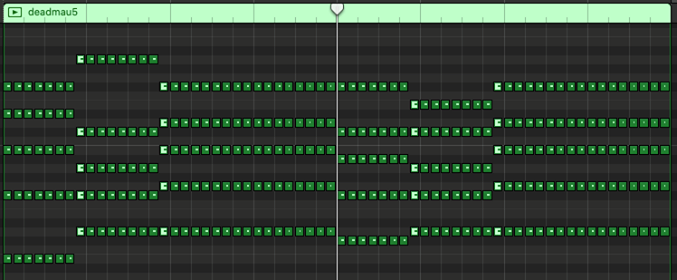The lengths of the chords are 7, 8 and 17. I had to convert this lengths to 8, 8 and 16. So I shifted the start, and put silence at the last beat.

``` TEST SI,1111000000000000B
JZ SKIP               ; no chords at the last beat
...                   ; chords here
DRUMS:
SUB SI,1000000000000B ; shifting back the drums by one beat
```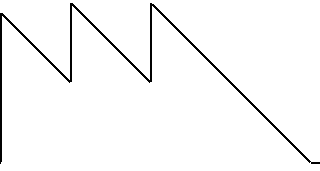But after it sucked at Revision, we've changed the music. I believe ern0 found much better chords. I prefer the new song's mood.

### Pattern

The stored data for musical notes is almost always shrinkable. The raw data is 32 bytes long:

```NOTES:
DB A_3,B_3,D_4,Fs4
DB B_3,D_4,E_4,Gs4
DB Cs4,E_4,Fs4,A_4
DB Cs4,E_4,Fs4,A_4

DB B_3,D_4,Fs4,B_4
DB B_3,Cs4,E_4,Gs4
DB Cs4,E_4,Fs4,A_4
DB Cs4,E_4,Fs4,A_4
```

Here is the simple decoder:

``` MOV DI,SI
SHRD DI,BX,2+16-5
AND DI,7*4
```

Look, we can save 12 bytes on the data...

```NOTES:
DB A_3,B_3,D_4,Fs4
DB B_3,D_4,E_4,Gs4

DB B_3,D_4,Fs4,B_4
DB B_3,Cs4,E_4,Gs4

DB Cs4,E_4,Fs4,A_4
```

and we lost only 7 bytes on the decoder.

``` MOV DI,4*4
SHR BL,1
JC .1
MOV DI,AX
SHLD DI,SI,3
AND DI,3*4
.1:
```

### Envelopes

We have two envelopes. Simple ADSR: no attack, just release.

Preparing CL and CH registers before the chord loop:

``` MOV AX,BX       ; BL: pattern
MOV CX,3
SHR AL,1
JC .1
MOV CH,128
.1:
DEC AX          ; skipping the drums-only intro
SHR AL,1
AND CL,AL
```

One envelope for tone...

``` IMUL AX,SI,-16
OR AL,111B
ROR AX,CL
MUL BX          ; DH: sample
```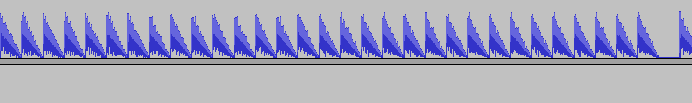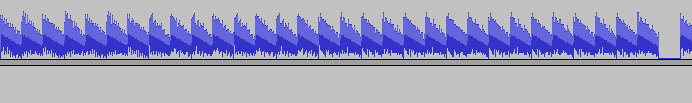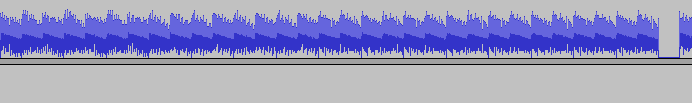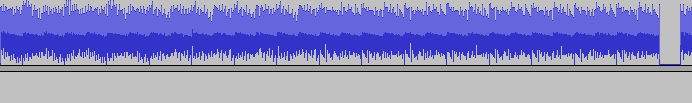and one for the volume...

``` IMUL AX,SI,-1
OR AH,CH        ; CH: 128 or 0
MUL DX          ; DH: sample
```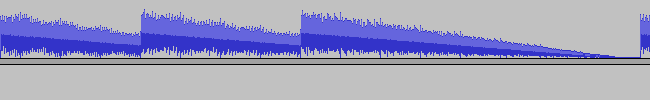### Mastering

After adding together every instrument, drum, and chord, I test the maximum volume. I always store the debug information to memory, and print it using an external util.

```if maxvol=1
CMP [FS:8000H],BH
JAE .1
MOV [FS:8000H],BH
.1:
end if
```

This time the maximum sample value was 181. So I will scale down to 7 bits only.

``` MOV AL,180       ; vol 181 -> vol 127
MUL BH           ; mastering
XCHG BX,AX
DONE:
MOV [BP+IRQ.SAMPLE-1],BH
```

But at the cutoff part, I skip this downscaling, and the chords become even louder.

Here is the whole generated song: